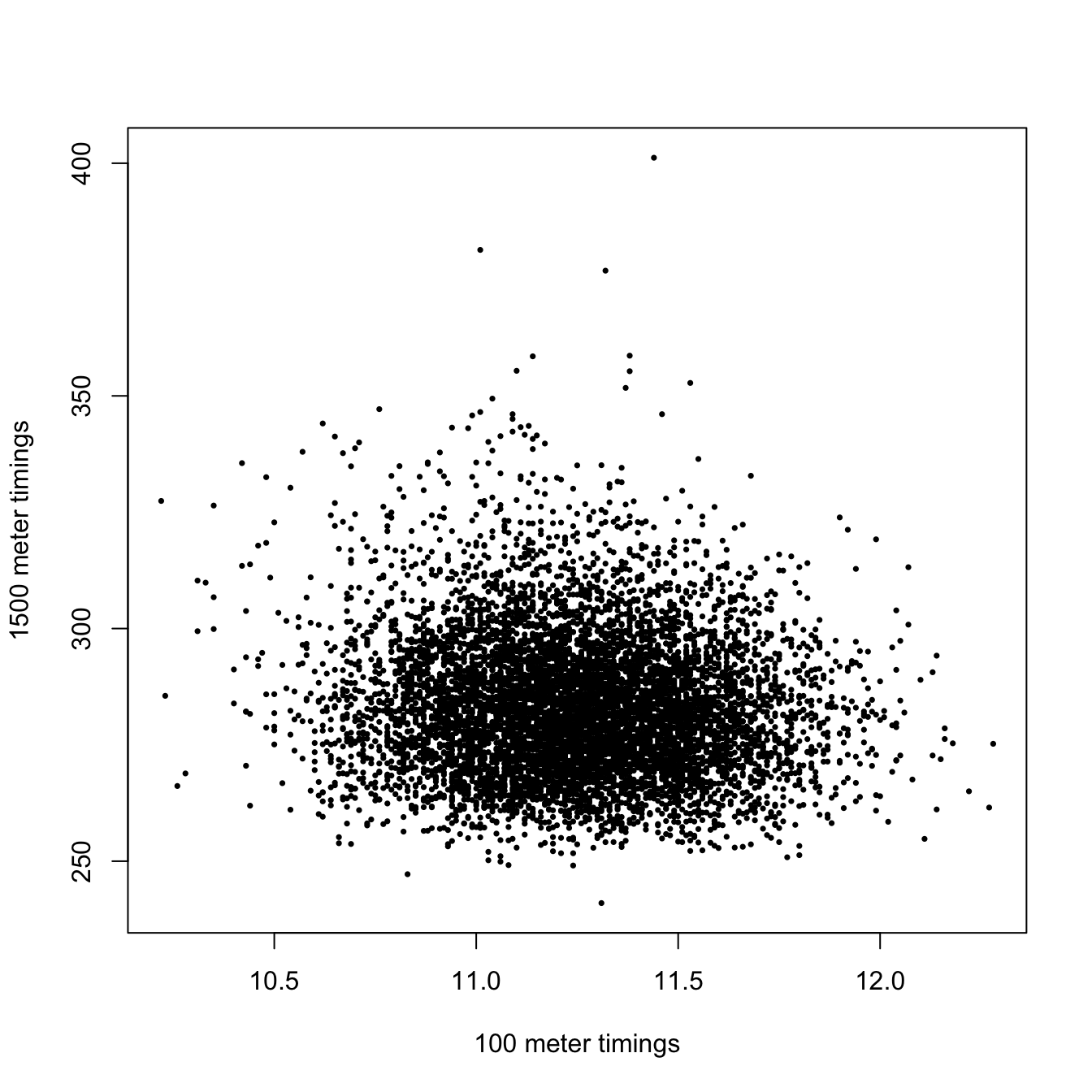Introduction

Outliers are observations within a dataset that seem not to belong with the rest of the data. They could be caused, for example, by spurious entries that need to be eliminated before further analysis, or by hard-to-detect signals of interest in their own right.

The probout package provides unsupervised estimates of the probability of outlyingness for observations, based largely on separation in terms of distance. It is intended for multivariate numeric data with large numbers of sequentially accessible observations. The dimensionality of the data should not be too large, so that distances between individual observations can be computed efficiently.

The method relies on leader clustering (Hartigan,1975) to reduce the size of the data in an initial phase. Leader clustering partitions the data into groups that are within a user-specified radius $$\rho$$ of leader observations. The leader observations are those that are not within $$\rho$$ of an existing leader as the data is processed sequentially. The leader observations, and hence the associated groups, will typically vary with the order of the data. By default, the data is normalized through min-max scaling, in which each variable is mapped to the unit interval.

After leader clustering, an outlier probability is determined for each group, based on the group centroids and data simulated from a mixture model defined by the group proportions, centroids, and variances, accumulated as the data is processed sequentially. The centroids are included to ensure representation of any groups with proportions so small that it would be unlikely that a simulated observation would be drawn from those groups.

Criteria for Outlyingness

probout estimates outlier probabilities by fitting an exponential distribution to a nonparametric outlier statistic from robust statistics (Stahel 1981, Donoho 1982). This statistic is essentially a robust $$z$$-score: for each observation, the median is subtracted and the absolute value of the result is divided by the median absolute deviation (MAD). For multivariate data, the univariate statistic is repeatedly computed for many random projections of the data, and the maximum value is retained as the value of the multvariate statistic. Outliers correspond to unusually large values of the outlier statistic.

Example

Example data

We use the 100, 400, 1500 meter timings from the Decathlon dataset from CRAN package GDAdata.

data(Decathlon)

x <- Decathlon[,c("m100","m400","m1500")]

A projection of the data onto the first and third coordinates can be produced as follows:

plot(x[,1], x[,3], xlab = "100 meter timings", ylab = "1500 meter timings",
main = "", pch = 16, cex = .5)The leader function produces a list of leader clusterings for each radius supplied as a argument. The default is to compute the leader clustering for a single radius, which corresponds to the default radius $$0.1 ~ / ~ log(n)^{(1/p)}$$ from Wilkinson (2016) — the same as in the HDoutliers package (Fraley 2016). A plot of the leaders can be produced as follows: